Courses

# Quantitative Aptitude - Test 8

## 25 Questions MCQ Test SSC CGL Tier 1 Mock Test Series | Quantitative Aptitude - Test 8

Description
This mock test of Quantitative Aptitude - Test 8 for SSC helps you for every SSC entrance exam. This contains 25 Multiple Choice Questions for SSC Quantitative Aptitude - Test 8 (mcq) to study with solutions a complete question bank. The solved questions answers in this Quantitative Aptitude - Test 8 quiz give you a good mix of easy questions and tough questions. SSC students definitely take this Quantitative Aptitude - Test 8 exercise for a better result in the exam. You can find other Quantitative Aptitude - Test 8 extra questions, long questions & short questions for SSC on EduRev as well by searching above.
QUESTION: 1

### A kite is flying at a height of 80 m from the level ground, attached to a string inclined at 30° to the horizontal. The length of the string is?

Solution: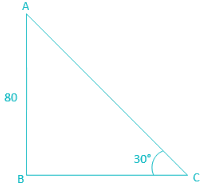From the diagram,
Height of the kite = AB = 80 m
Angle of inclination of the string with horizontal = ∠ ACB = 30°
Length of the string = AC
From ΔABC,
sin ∠ACB = Perpendicular/Hypotenuse
⇒ sin 30° = AB/AC
⇒ 1/2 = 80/AC
⇒ AC = 160 m

QUESTION: 2

### If tan θ + cot θ = 2, then the value of tannθ + cotnθ (0° < θ < 90°, n is an integer) is

Solution:

tan θ  + cot θ = 2
∵ cot θ = 1/tan θ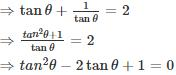Using the identity: a2 – 2ab + b2 = (a – b)2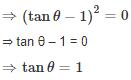∴ cot θ  = 1/tan θ  = 1/1 = 1
Given expression is,
tann θ  + cotn θ
= 1n + 1n
= 1+ 1 = 2

QUESTION: 3

### If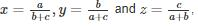then value of xy + yz + zx + 2xyz is

Solution:

We have to find the value of expression xy + yz + zx + 2xyz
Multiplying and dividing by xyz we have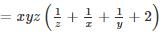Substituting values of x, y and z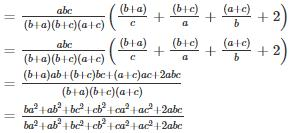= 1

QUESTION: 4

Find the coefficient of x2 in the expansion of (x2 + x + 1) (x2 – x + 1)

Solution:

We are given the following equation (x2 + x + 1) (x2 – x + 1)
Simplifying it, we get
= x4 - x3 + x2 + x3 - x2 + x + x2 – x + 1
= x4 + x+ 1
So, coefficient of xis 1

QUESTION: 5

Directions: Study the following Histogram and answer the following questions.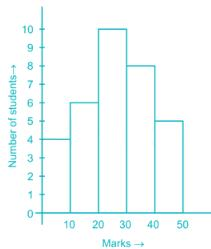Q. The ratio of the students obtaining marks in the first and the last interval is

Solution:

Number of students with marks 0 – 10 (first interval) = 4
Number of students with marks 40 – 50 (last interval) = 5
Required ratio = 4 : 5

QUESTION: 6

Directions: Study the following Histogram and answer the following questions.Q. The least number of students got the marks in the interval

Solution:

From the above graph, we can observe that
Number of students with marks 0 – 10 = 4
Number of students with marks 10 – 20 = 6
Number of students with marks 20 – 30 = 10
Number of students with marks 30 – 40 = 8
Number of students with marks 40 – 50 = 5
Hence, minimum numbers of students are in the interval 0 – 10 marks

QUESTION: 7

Directions: Study the following Histogram and answer the following questions.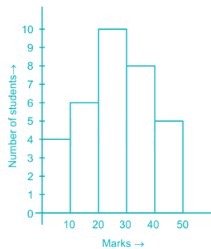Q. The maximum number of students got the marks in the interval of

Solution:

From the above graph, we can observe that
Number of students with marks 0 – 10 = 4
Number of students with marks 10 – 20 = 6
Number of students with marks 20 – 30 = 10
Number of students with marks 30 – 40 = 8
Number of students with marks 40 – 50 = 5
Hence, maximum numbers of students are in the interval 20 – 30 marks

QUESTION: 8

Directions: Study the following Histogram and answer the following questions.Q. The total number of students involved in the data is

Solution:

From the above graph, we can observe that
Number of students with marks 0 – 10 = 4
Number of students with marks 10 – 20 = 6
Number of students with marks 20 – 30 = 10
Number of students with marks 30 – 40 = 8
Number of students with marks 40 – 50 = 5
Total students = 4 + 6 + 10 + 8 + 5

= 33

QUESTION: 9

The intersecting point of the graphs x – y = 0 and x + y – 4 = 0 is

Solution:

Given,
Equations are
X – y = 0 ……(1)
x + y – 4 = 0
x + y = 4 ……(2)
By solving these two equations we will get the point of intersection
Now,
(1) + (2)
x – y + x + y = 0 + 4
⇒ 2x = 4
⇒ x = 2
Putting this value in (1)
2 – y = 0
⇒ y = 2
∴ Point of intersection is (2, 2)

QUESTION: 10

If sides AB, BC and the median AE in ΔABC is similar to the sides PQ, QR and median PO in ΔPQR. State whether ΔABC is similar to, ΔPQR?

Solution: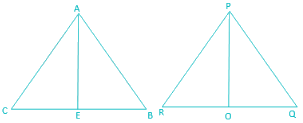Given, AB, BC and the median AE in ΔABC is similar to the sides PQ, QR and median PO in ΔPQR.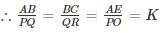AE and PO are medians, EB = EC and OQ = OR
∵ AE and PO are medians, EB = EC and OQ = OR
∴ Triangles ABE and PQO are similar by SSS as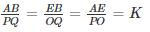By property of similar triangles, ∠ABC = ∠PQR
∴ Triangles ABC and PQR are similar by SAS as,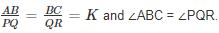QUESTION: 11

If AD is the median of the triangle ABC and G be the centroid, then the ratio of AG:AD is

Solution:

If AD is the median of the triangle ABC and G be the centroid then we know that
AG:GD = 2:1
Now adding the numerator in the denominator we get,
⇒ AG : (AG + GD) = 2: (2 +1)
⇒ AG : AD = 2:3

QUESTION: 12

Let the lengths of the sides of a triangles be represented by x + 3, 2x – 3 and 3x – 5. If the perimeter of the triangle is 31, then the length of the longest side is

Solution:

Perimeter of a triangle = Sum of all sides
Given, length of the sides of a triangles be represented by x + 3, 2x – 3 and 3x – 5.
Perimeter = 31
⇒ x + 3 + 2x – 3 + 3x – 5 = 31
⇒ 6x = 36
⇒ x = 6
Sides are 9, 9 and 13
Thus, Longest side = 13

QUESTION: 13

At each corner of a triangular field of sides 26 m, 28 m and 30 m, a cow is tethered by a rope of, length 7 m. The area (in m2) ungrazed by the cows is

Solution:

We know that area of arc of a circle = ½ × r2 × θ
Where θ is the angle traced by the arc in radians.
Since the length of the rope is same for all three cows thus the radius of the arc traced by them is same i.e. 7m (given).
Also total angle traced by all three cows together is 180° as it is a triangular field.
Hence, total are grazed by 3 cows together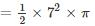= 77 m2
Now, Area of triangular field by using heron’s formula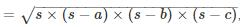where s is the semi perimeter of the triangle
Given sides of triangle are 26m, 28m and 30m.
Hence, semi perimeter, s = (26 + 28 + 30)/2
= 42 m
∴ Area of triangular field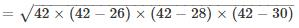= 336 m2
Hence, the area of ungrazed field
= Area of triangular field – total area grazed by 3 cows
= 336 – 77
= 259 m2

QUESTION: 14

The radii of the two ends of a frustum are 20 cm and 13 cm respectively. If the height of the frustum is 24 cm, then its slant height is

Solution: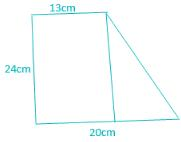Pythagoras theorem:-
Hypotenuse2 = perpendicular2 + base2
The figure represents the side view of a frustum.
Perpendicular = 24cm
Base = 20 – 13 = 7cm
Hypotenuse = Slant height.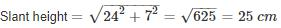QUESTION: 15

Raman takes twice time as much as Ajay to do a work. If they work together, the work is completed in 12 days. How many days will Raman take to complete the work alone?

Solution:

Let the number of days in which Ajay finishes the work be ‘d’ days.
Raman takes twice time, thus number of days in which Raman finishes the work = 2d days.
In 1 day, Ajay finishes 1/d part of the work while Ajay finishes 1/2d part of the work
Working together, in one day, they finish part of work = 1/d + 1/2d = 3/2d
∴ Number of days in which they finish the work = 2d/3
Given, they take 12 days to finish the work.
∴ 2d/3 = 12
⇒ d = 18 days
∴ Number of days Raman will take = 2d = 36 days

QUESTION: 16

A bus rides at the rate of 48 kms/hr and stops for 18 mins to change course after every 18kms. Total time taken by him to cover a distance of 288 km is –

Solution:

Given,
Total distance = 288 km
Speed = 48 kms/hr
Time taken to travel 288 km = Distance/Speed= 288/48 = 6 hour
Now we need to find out the number of stops in the 288 km travel. Given that train stops after every 18 km.
∴ Number of stops = 288/18 = 16
It means train stops 15 times before 288 km and 1 time just after 288 km.
∴ We need to take only 15 stops into consideration for the 288 km travel.
∴ Total stopping time in the 288 km travel = 15 × 18 = 270 minutes
∴ Total time needed to reach the destination
= 6 hours + 270 minutes
= 6 hours + 4 hours + 30 minutes
= 10 hours 30 minutes

QUESTION: 17

Mayur is walking at a speed of 15 km per hour. After every km, he takes rest for 6 minutes. How much time will he take to cover a distance of 11 km?

Solution:

We have Mayur covering a distance of 15 km in 1 hour.
Hence time taken to complete 1 km =
As after every 1 km, he Mayur takes a rest for 6 minutes, effective time to finish 1 km = 4 + 6 = 10 min
To cover first 10 km, he needs = 10 × 10 = 100 mins
For the last 1 km, he need = 4 min (he will not take time to rest as he has reached the finish point)
Total time needed = 100 + 4 = 104 min
Or 104/60 hours
= 1 hour and 44 minutes

QUESTION: 18

A fruit seller purchased 50 kg of mangoes at the rate of Rs. 25 per kilo. He sold some of the mangoes at the rate of Rs. 40 per kilo and the rest at the rate of Rs. 15 per kilo. If he incurred 20% profit overall, the amount of mangoes sold at the rate of Rs. 15 per kilo is

Solution:

A fruit seller purchased 50 kg of mangoes at the rate of Rs. 25 per kilo.
Total cost price of the 50 kg of mangoes = 50 × 25 = 1250
If he incurred 20% profit overall then the selling price of 50 Kg of Mangoes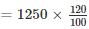= 1500
He sold some of the mangoes at the rate of Rs. 40 per kilo and the rest at the rate of Rs. 15 per kilo.
Suppose he sold X kg of mangoes at the rate of Rs 40 and (50-X) Kg of sold at the rate of Rs15.
We can say that,
⇒ 40 × X + 15 × (50 - X) = 1500
⇒ 40X + 750 – 15X = 1500
⇒ 25X = 750
⇒ X = 30
So he sold 30 kg of mangoes at the rate of Rs 40 and (50-30) =20 Kg of sold at the rate of Rs 15.
Hence the answer is 20 kg

QUESTION: 19

A shopkeeper gives 12% additional discount on the discounted price after giving an initial discount of 20 % on the labeled price of a radio. If the final sale price is Rs. 3520, then what is its labeled price?

Solution:

Formula: Let the successive increase in percentages be a% and b%.
In that case, the total increase will be (a + b + ab/100) %
A shopkeeper gives 12% additional discount on the discounted price after giving an initial discount of 20 % on the labeled price of a radio.
So here a= - 12 and b = - 20
The total successive discount = a + b + ab/100
= (-12) + (-20) + (-12) × (-20)/100
= -12 – 20 + 2.4
= - 29.6
Hence if the Label price is 100 then after giving 29.6% discount the final sale price is (100 -29.6) = 70.4
So if the final sale price is Rs 70.4 then the label price is Rs 100
If the final sale price is Rs 1 then the label price is Rs 100/70.4
If the final sale price is Rs 3520 then the label price is Rs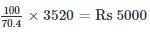Hence the answer is Rs 5000.

QUESTION: 20

The average age of the boys in a class of 20 boys is 15.6 years. What will be the average age if 5 new boys come whose average is 15.4 years?

Solution:

We have, Average age = Sum of all ages/No. of persons
∴ 15.6 = Sum of 20 boys ages/ 20
⇒ Sum of ages of 20 boys = 20 × 15.6 = 312
After 5 new boys come, total boys = 20 + 5 = 25
Sum of ages of 5 new boys = 15.4 × 5 = 77
Sum of 25 boys ages = 312 + 77 = 389
Overall average = 389/25 = 15.56 yrs.

QUESTION: 21

The price of a commodity rises from Rs. 6 per kg to Rs. 7.50 per kg. If the expenditure cannot increase, the percentage of reduction in consumption is

Solution:

Given, price of a commodity rises from Rs. 6 per kg to Rs. 7.50 per kg.
Also given, the expenditure remains the same.
Thus, initial consumption × 6 = New consumption × 7.5
⇒ New consumption = 0.8 × initial consumption
Decrease in consumption = Initial consumption – Final consumption
⇒ Decrease in consumption = Initial consumption - 0.8 × initial consumption
⇒ Decrease in consumption = 0.2 × initial consumption

% decrease in consumption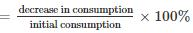⇒ % decrease in consumption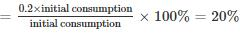QUESTION: 22

Neena borrows Rs. 1500. The rate of simple interest is same as the number of years the money has been borrowed for. If she gives Rs. 540 as interest at the end of the stipulated period, the rate of interest p.c.p.a. will be

Solution:

Let the No. of yrs for which the loan has been taken = x yrs
According to question Rate of Interest Applicable = x% pa
We know that, formula for simple interest: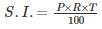Where,
S.I. = Simple Interest = 540
P= principal = 1500
T = Time = x
R = Rate of Interest = x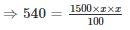⇒ 540 = 15x2
⇒ x2 = 36
Taking Square root on both sides
⇒ x = 6
⇒ Percent Per Annum (PCPA) = 6%

QUESTION: 23

An alloy contains copper, zinc and nickel in the ratio of 5:3:2. The quantity of nickel in kg that must be added to 100 kg of this alloy to have the new ratio 5:3:3 is

Solution:

Let X kg of nickel be added to the alloy.
Given, Initial ratio is 5:3:2.
Let the initial weight of copper, zinc and nickel in 100 kg of alloy be 5Y, 3Y and 2Y respectively.
Also, initial weight of alloy = 100 kg
∴ 5Y + 3Y + 2Y = 100 kg
⇒ Y = 10
Since X kg of nickel is added to the alloy, thus
⇒ New weight of nickel = X + 20 kg
Given in question, new ratio after this addition is 5:3:3. On comparing it with the old ratio 5:3:2 we find that the weight of nickel has increased by 10 kg i.e
New weight of nickel = 20 + 10
= 30 kg
∴ X + 20 = 30
⇒ X = 10 kg
Hence, 10 kg of nickel must be added to the alloy to get new ratio of 5:3:3.

QUESTION: 24

Four identical circles of radius 4 cm each touch each other externally. The area of the region bounded by the four circles (in cm2) is

Solution: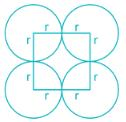Area of a square = side2
Area of a circle = πr2
The radius of each circle in the figure (r) = 4 cm.
We have constructed a square by joining the centers of 4 circles.
∴ Each side of the square = (4+4) cm. = 8 cm.
Now, if we consider any one of the circles it is clearly visible that the square includes ¼ th of each circle.
Now total area of all circles included inside the square
= 4 × [¼ × area of one circle]
= area of one circle
= 16 × �� cm2 [∵ area of circle = �� × r2]
The area of the square = 82 = 64 cm2
From the figure, the area of the region bounded by the four circles = the area of the square - total area of all circles included inside the triangle
⇒ The area of the region bounded by the four circles:
= [64 - 16 × ��] cm.2
= 16 × [4 – ��] cm.2

QUESTION: 25

From each corner of a square of side 4 cm, a quadrant of a circle of radius 1 cm is cut and also a circle of diameter 2 cm is cut as shown is figure. The area of the remaining (shaded) portion is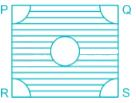Solution:

Area of a square = side × side
Area of a circle = πr2,
Area of quadrant of a circle = πr2/4